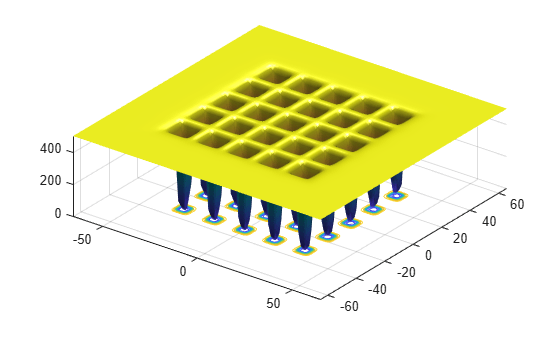# Optimize Using Particle Swarm

This example shows how to optimize using the `particleswarm` solver.

The objective function in this example is De Jong’s fifth function, which is available when you run this example.

`dejong5fcn`This function has 25 local minima.

Try to find the minimum of the function using the default `particleswarm` settings.

```fun = @dejong5fcn; nvars = 2; rng default % For reproducibility [x,fval,exitflag] = particleswarm(fun,nvars)```
```Optimization ended: relative change in the objective value over the last OPTIONS.MaxStallIterations iterations is less than OPTIONS.FunctionTolerance. ```
```x = 1×2 -31.9521 -16.0176 ```
```fval = 5.9288 ```
```exitflag = 1 ```

Is the solution `x` the global optimum? It is unclear at this point. Looking at the function plot shows that the function has local minima for components in the range `[-50,50]`. So restricting the range of the variables to `[-50,50]` helps the solver locate a global minimum.

```lb = [-50;-50]; ub = -lb; [x,fval,exitflag] = particleswarm(fun,nvars,lb,ub)```
```Optimization ended: relative change in the objective value over the last OPTIONS.MaxStallIterations iterations is less than OPTIONS.FunctionTolerance. ```
```x = 1×2 -16.0079 -31.9697 ```
```fval = 1.9920 ```
```exitflag = 1 ```

This looks promising: the new solution has lower `fval` than the previous one. But is `x` truly a global solution? Try minimizing again with more particles, to better search the region.

```options = optimoptions('particleswarm','SwarmSize',100); [x,fval,exitflag] = particleswarm(fun,nvars,lb,ub,options)```
```Optimization ended: relative change in the objective value over the last OPTIONS.MaxStallIterations iterations is less than OPTIONS.FunctionTolerance. ```
```x = 1×2 -31.9781 -31.9784 ```
```fval = 0.9980 ```
```exitflag = 1 ```

This looks even more promising. But is this answer a global solution, and how accurate is it? Rerun the solver with a hybrid function. `particleswarm` calls the hybrid function after `particleswarm` finishes its iterations.

```options.HybridFcn = @fmincon; [x,fval,exitflag] = particleswarm(fun,nvars,lb,ub,options)```
```Optimization ended: relative change in the objective value over the last OPTIONS.MaxStallIterations iterations is less than OPTIONS.FunctionTolerance. ```
```x = 1×2 -31.9783 -31.9784 ```
```fval = 0.9980 ```
```exitflag = 1 ```

`particleswarm` found essentially the same solution as before. This gives you some confidence that `particleswarm` reports a local minimum and that the final `x` is the global solution.